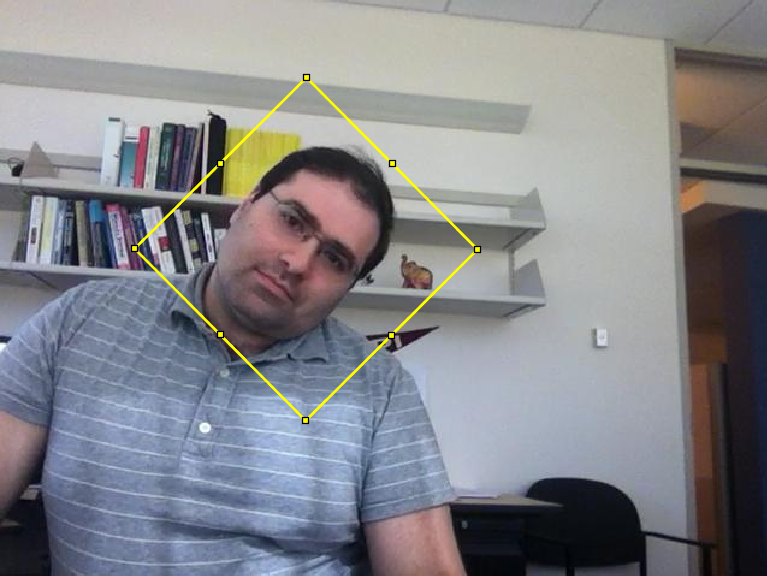# Minimally Bounded Rectangles, and more!

Brett‘s Pick this week is A suite of minimal bounding objects, by John D’Errico. I also give a shout-out to julien diener, for his 2D minimal bounding box function.

During the course of developing some code to automatically track objects in videos, I found that I needed to create a “minimal bounding rectangle” to enclose detected points. There is a very nice demo in the Computer Vision Toolbox in which points used to track a moving face are delineated by a polygon created by the polyshape command. All well and good; it works nicely, and is very fast. But for other reasons, I preferred to work with an ‘images.roi.Rectangle’ object, as created by the drawrectangle command (which is itself a convenience function for rectangle().)

A modified version of the aforementioned example looks like this:

vidObj = VideoReader('tilted_face.avi');
togglefig('Face Tracker')
imgH = imshow(videoFrame);
bbox = faceDetector(videoFrame);
bboxPoints = bbox2points(bbox(1, :));
thisPoly = polyshape(bboxPoints);
hold on
polyH = plot(thisPoly, 'FaceAlpha', 0, 'EdgeColor', 'y', 'LineWidth', 2);
points = detectMinEigenFeatures(rgb2gray(videoFrame), 'ROI', bbox);
pointTracker = vision.PointTracker('MaxBidirectionalError', 2);
points = points.Location;
initialize(pointTracker, points, videoFrame);
oldPoints = points;
tic;
while hasFrame(vidObj)
% Get the next frame, update the visualization:
imgH.CData = videoFrame;
% Track the points. Note that some points may be lost.
[points, isFound] = pointTracker(videoFrame);
visiblePoints = points(isFound, :);
oldInliers = oldPoints(isFound, :);
if size(visiblePoints, 1) >= 2 % need at least 2 points
[xform, oldInliers, visiblePoints] = estimateGeometricTransform(...
oldInliers, visiblePoints, 'similarity', 'MaxDistance', 4);
bboxPoints = transformPointsForward(xform, bboxPoints);
thisPoly = polyshape(bboxPoints);
polyH.Shape = thisPoly;
oldPoints = visiblePoints;
setPoints(pointTracker, oldPoints);
end
imgH.CData = videoFrame;
drawnow
end
t = toc;
% Clean up
release(pointTracker);

% (Processing the while loop takes about 6.5 seconds on my laptop.)But how would one replace the polygon with a rotated rectangle? Fortunately for us, there's the File Exchange, where a search for "minimal bounding rectangle" quickly turned up John's "suite" of functions for minimally bounding circles, spheres, parallelograms, quadrilaterals, semicircles, triangles, and rectangles.As we've come to expect, John's functions are well written and commented. It was a simple matter to replace the polygon with a minimally bounded rectangle, which not surprisingly computes the convex hull of the input points. One note: John's function returned the x- and y- coordinates of the target rectangle, and the area and perimeter of the same:[rectx,recty,area,perimeter] = minboundrect(x,y,metric)

(In fact, one can elect, using the 'metric' parameter, to minimize by area or perimeter, with area being the default.) I needed the rotation angle of the triangle, to use as an input in drawrectangle. It took a moment to modify minboundrect to return as an additional output the edgeangles parameter, which is computed internally. In moments, we had:
vidObj.CurrentTime = 0; % Reset and reuse the VideoReader object
%togglefig('Face Tracker', true) %Clear and reuse figure
%imgH = imshow(videoFrame);
bbox = faceDetector(videoFrame);
% hRect = drawrectangle('Position', bbox, ...
%     'FaceAlpha', 0, 'Color', 'y', 'LineWidth', 2);
bboxPoints = bbox2points(bbox(1, :));
points = detectMinEigenFeatures(rgb2gray(videoFrame), 'ROI', bbox);
pointTracker = vision.PointTracker('MaxBidirectionalError', 2);
points = points.Location;
initialize(pointTracker, points, videoFrame);
oldPoints = points;
tic;
while hasFrame(vidObj)
% Get the next frame, update the visualization:
%     imgH.CData = videoFrame;
% Track the points. Note that some points may be lost.
[points, isFound] = pointTracker(videoFrame);
visiblePoints = points(isFound, :);
oldInliers = oldPoints(isFound, :);
if size(visiblePoints, 1) >= 2 % need at least 2 points
[xform, oldInliers, visiblePoints] = estimateGeometricTransform(...
oldInliers, visiblePoints, 'similarity', 'MaxDistance', 4);
bboxPoints = double(transformPointsForward(xform, bboxPoints));
% |vertToPos| is a helper function I wrote; it is the inverter for
% <https://www.mathworks.com/help/vision/ref/bbox2points.html |bbox2points|>.
test = 'john';
switch test
case 'john'
[rectx, recty, theta] = ...
minboundrect(bboxPoints(:,1), bboxPoints(:,2));
rectPos = vertToPos([rectx, recty]);
case 'julien'
[rect, theta] = minBoundingBox(bboxPoints');
rectPos = vertToPos(rect');
end
hRect.Position = rectPos;
hRect.RotationAngle = theta;
oldPoints = visiblePoints;
setPoints(pointTracker, oldPoints);
end
%     imgH.CData = videoFrame;
drawnow
end
t = toc;
% Clean up
release(pointTracker);

Looping over all the video frames took 8.3 seconds; minboundrect added about 4 msec per frame overhead. (Not bad!).
As a side note, I also found my way to (and tested--see the switch-case in the code above) julien's '2D minimal bounding box' contribution. julien (who apparently eschews capitalization) wrote that his code is "fully vectorized," and thus "is better for big set of points." I didn't test the difference on a large dataset, but for my use case, I could detect no performance difference. Very nice, gentlemen--thank you both!
As always, I welcome your thoughts and comments.
<!--
// Remember the title so we can use it in the new page
title = document.title;

// Break up these strings so that their presence
// in the Javascript doesn't mess up the search for
// the MATLAB code.
t1='36c6418b22fc4315ade02fb81935013d ' + '##### ' + 'SOURCE BEGIN' + ' #####';
t2='##### ' + 'SOURCE END' + ' #####' + ' 36c6418b22fc4315ade02fb81935013d';

b=document.getElementsByTagName('body');
i1=b.innerHTML.indexOf(t1)+t1.length;
i2=b.innerHTML.indexOf(t2);

code_string = b.innerHTML.substring(i1, i2);
code_string = code_string.replace(/REPLACE_WITH_DASH_DASH/g,'--');

// Use /x3C/g instead of the less-than character to avoid errors
// in the XML parser.
// Use '\x26#60;' instead of '<' so that the XML parser
// doesn't go ahead and substitute the less-than character.
code_string = code_string.replace(/\x3C/g, '\x26#60;');

copyright = 'Copyright 2020 The MathWorks, Inc.';

w = window.open();
d = w.document;
d.write('

<pre>\n');
d.write(code_string);

// Add copyright line at the bottom if specified.
if (copyright.length > 0) {
d.writeln('');
d.writeln('%%');
if (copyright.length > 0) {
d.writeln('% _' + copyright + '_');
}
}

d.write('</pre>

\n');

d.title = title + ' (MATLAB code)';
d.close();
}
-->

Published with MATLAB® R2019b


|

### コメント

コメントを残すには、ここ をクリックして MathWorks アカウントにサインインするか新しい MathWorks アカウントを作成します。